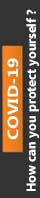We're Open

0% Plagiarism Guaranteed & Custom Written

# Question 1

The circle has a radius of r metres and the longer circular arc joining A and B is two times as long as the chord AB. Find the length of the chord AB, correct to 6 d.p. (Hint: Use NewtonRaphson).

Question 2

An ancient walled structure, that is assumed to be circular in plan, is required to be surveyed.

Due to its structural instability, it is not considered safe to go inside, or near the structure, and so two survey points P and Q have been established such that P is A metres north of the most Northerly part of the structure, and Q is B metres east of the most Southerly part of the structure. The points P and Q have been carefully chosen so that the point Q is just visible from point P. (i.e. the line of sight between the two points is tangential to the wall of the structure.

Use this information to find the diameter of the structure by constructing and solving a nonlinear equation.

# Question 3

Using the Euler Numerical Method, solve the following problems:

1. The maximum displacement (y mm) of a prestressed concrete beam at a distance x (m) is  given by the equation: -

𝑑𝑑𝑑𝑑       2+𝐵𝐵𝑑𝑑2

=𝐴𝐴𝑑𝑑

𝑑𝑑𝑑𝑑

If the initial conditions are x0 =0 , and yo = 0, find the maximum value that x can have if the maximum permitted displacement is Cmm.

1. A differential equation for relating the spread of a contaminant in a ground contamination investigation is given as:-

𝑑𝑑𝑑𝑑 𝐷𝐷𝑑𝑑+𝑑𝑑

=

𝑑𝑑𝑑𝑑     𝑑𝑑𝑑𝑑

Initially, x = 1, and y =2, find the value of y when x = E.

100% Plagiarism Free & Custom Written,International House, 12 Constance Street, London, United Kingdom,
E16 2DQ

## STILL NOT CONVINCED?

We've produced some samples of what you can expect from our Academic Writing Service - these are created by our writers to show you the kind of high-quality work you'll receive. Take a look for yourself!FLAT 25% OFF ON EVERY ORDER.Use "FLAT25" as your promo code during checkout